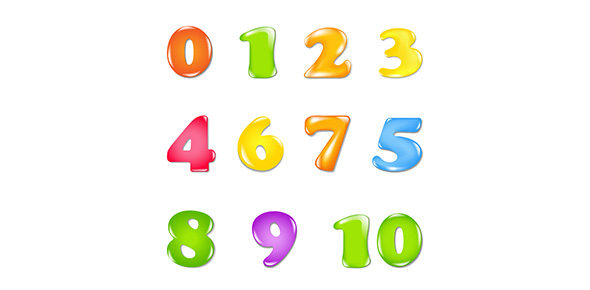# Multiplication Of Whole Numbers

7 Questions | Total Attempts: 575SettingsThis quiz is used as part of an eLearning program. If you can pass it, you don't need to proceed with the rest of the multiplication tutorial.

• 1.
Which of the following symbols represents multiplication?
• A.

+

• B.

-

• C.

X

• D.

÷

• E.

=

• 2.
To multiply means to add up groups of objects. True or False?
• A.

True

• B.

False

• 3.
Solve this multiplication question using a pencil and paper:(no calculators allowed)7 X 6 =
• 4.
Solve this multiplication question using a pencil and paper:(no calculators allowed)8 X 19 =
• 5.
Solve this multiplication question using a pencil and paper:(no calculators allowed)73 X 45 =
• 6.
Solve this multiplication question using a pencil and paper:(no calculators allowed)62 X 104 =
• 7.
Solve this multiplication question using a pencil and paper:(no calculators allowed)370 X 8806 =
Related TopicsBack to top